# Configuring a DC Sweep Analysis in Multisim

Publish Date: Mar 27, 2012 | 7 Ratings | 2.29 out of 5 | Print | Submit your review

## Overview

Multisim features a comprehensive suite of SPICE analyses for examining circuit behavior. These analyses range from the basic to sophisticated. Each analysis helps you to obtain valuable information such as the effects of component tolerances and sensitivities. For each analysis you need to set settings that will inform Multisim exactly what to analyze, and how.

Multisim simplifies the procedure for an advanced analysis by providing a configuration window. This abstracts away the complexities associated with SPICE syntax and configuration of an analysis. With this window you merely need to specify the parameter values and output nodes of interest.

This tutorial is part of the National Instruments SPICE Analysis Fundamentals Series. Each tutorial in this series provides you with step-by-step instructions on how to configure and run the different SPICE analyses available in Multisim.powerful simulation and analysis while abstracting the complexity of SPICE syntax.

### 1. Introduction

DC Sweep Analysis is used to calculate a circuits’ bias point over a range of values. This procedure allows you to simulate a circuit many times, sweeping the DC values within a predetermined range. You can control the source values by choosing the start and stop values and the increment for the DC range. The bias point of the circuit is calculated for each value of the sweep.

Multisim performs DC Sweep Analysis using the following process:

1. The DC Operating Point is calculated using a specified start value.
2. The value from the source is incremented and another DC Operating Point is calculated.
3. The increment value is added again and the process continues until the stop value is reached.
4. The result is displayed on the Grapher View.

Assumptions: Capacitors are treated as open circuits, inductors as shorts. Only DC values for voltage and current sources are used.

### 2. Running DC Sweep Analysis

In this section you will use two example circuits to learn how to configure DC Sweep Analysis.

Example 1:

1. Open circuit file zener.ms11 located in the Downloads section.  This is a simple Zener regulator circuit where the diode maintains a nearly constant output even though the current through it changes.

In this case, the 1N4462 component has a Zener voltage (VZ) of 7.5 V and a 5% tolerance. The minimum and maximum input voltages that can be regulated by the Zener diode are 7.41 V and 26.41 V respectively. You will use DC Sweep Analysis to study the behavior of the regulator circuit when the input voltage is varied from 0 V to 40 V.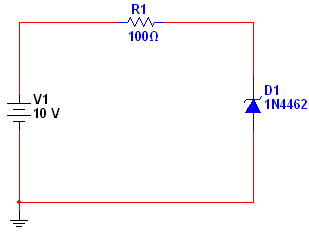Figure 1. Zener regulator.

1. Select Simulate»Analyses»DC Sweep. The DC Sweep Analysis window opens.Table 1 describes the Analysis Parameters tab in detail.

Table 1. Parameters used in DC Sweep Analysis.

 Parameter Meaning Source Lists all independent sources (voltage or current) in the circuit. Start Value Starting value of the sweep. Stop Value Ending value of the sweep. Increment Value by which each sweep will increase. Change Filter Filters the variables displayed to include internal nodes (such as nodes inside a BJT model or inside a SPICE subcircuit), open pins, as well as output variables from any sub-modules contained in the circuit.

Note: In SPICE, the command that performs an DC Sweep Analysis has the following form:

.DC <SOURCE_NAME> <START_VALUE> <STOP_VALUE> <INCREMENT>

Basically these are the same parameters that were defined in Table 1 but in Multisim you do not have to worry about the SPICE syntax.

1. Configure the Analysis Parameters as shown in Figure 2.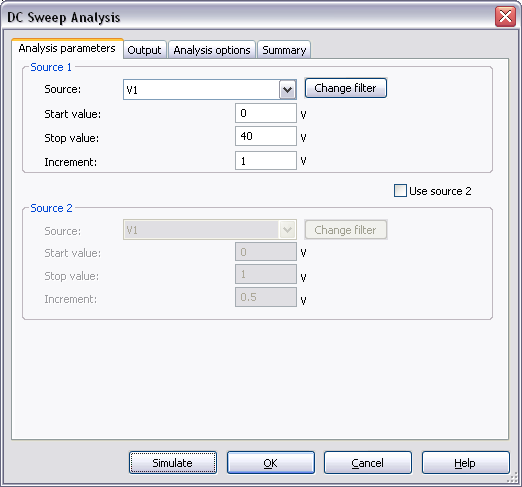Figure 2. Analysis parameters for the DC Sweep Analysis.

As you can see, V1 will be vary from 0 to 40 V with increments of 1 V. Note that you can add a second source to the analysis by enabling the Use source 2 option.  This is useful to perform a nested sweep (see Example 2).

1. Select the Output tab.
2. Select the Variables in circuit list, select All variables from the drop-down list, and then highlight V(out) from the list.
3. Click the Add button to move the variable to the right side under Selected variables for analysis, as shown below.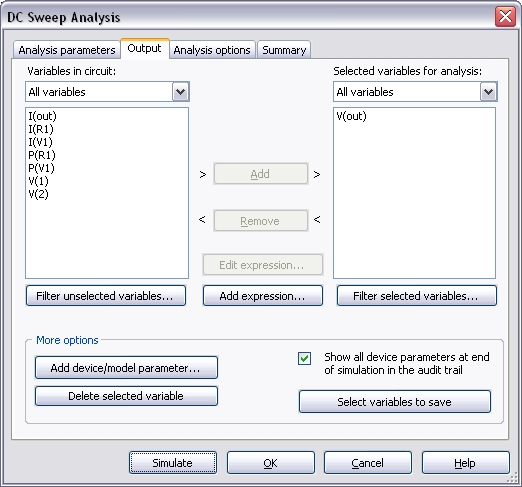Figure 3. Output variable for the DC Sweep Analysis.

1. Click Simulate. The Grapher View window opens. Result is displayed in Figure 4.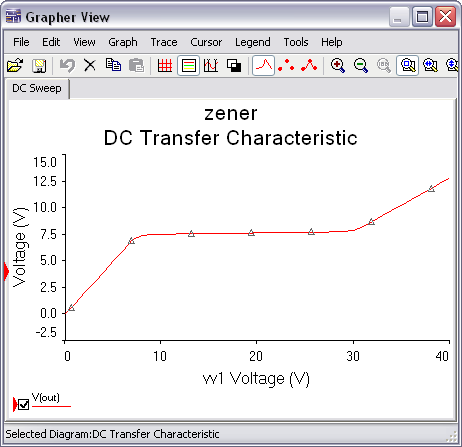Figure 4. DC Sweep Analysis results.

From the Grapher View you can observe that the output voltage steadies at around 7.5 V, which is the nominal Zener voltage for the 1N4462. Use the cursors to calculate the minimum and maximum voltages that this component can regulate.

Example 2:

1. Open the file mosfet_curve.ms11 (from Downloads). This is a simple circuit where you will use DC Sweep Analysis to obtain the MOSFET characteristic curves.

V1 and V2 (from Figure 5) need to be swept to obtain the characteristic curves.  Note that in order to convert the current flow through the drain to a voltage (for display on the Grapher View), a current controlled voltage source (V3) has been added to the circuit.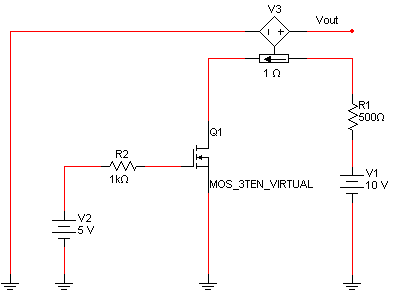Figure 5. MOSFET circuit.

1. Select Simulate»Analyses»DC Sweep. The DC Sweep Analysis window opens.
2. Configure the Analysis Parameters as shown in the following figure: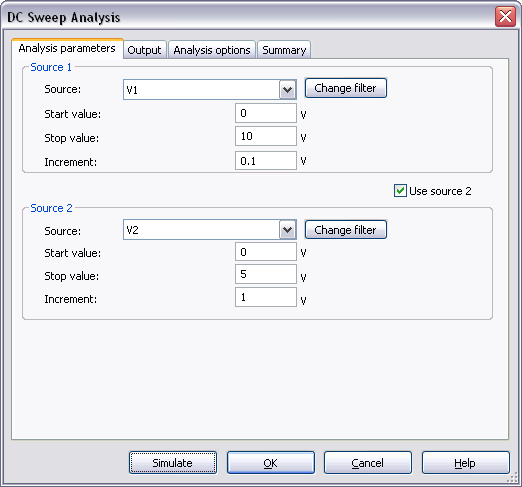Figure 6. Analysis parameters for the nested DC sweep.

Note that a second sweep variable (V2) has been added so that the analysis can perform a nested sweep. The first sweep (V1) will be done for each value of the second sweep. The number of output curves will be equal to the number of points for the second source.

In this example V2 will be set to 0 V (the Start value) and then V1 will be sweep from 0 V to 10 V; this will generate the first curve. In the next loop V2 will be set to 1 V and V1 will be sweep again from 0 V to 10 V generating the second curve. This process will continue until the Stop value for V2 is reached.

1. Select the Output tab and make sure that V(vout) is listed under Selected variables for analysis.
2. Click Simulate. Results will be displayed in the Grapher View.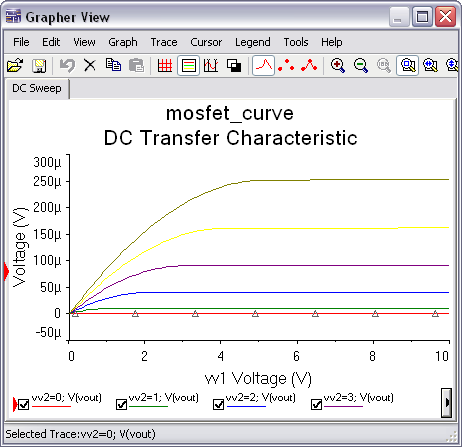Figure 7. DC Sweep Analysis results.

In Multisim, you can also generate current-voltage curves using the IV Analyzer instrument.

Entering Expressions in Analyses in Multisim

SPICE Analysis Fundamentals

Circuit Design Technical Library

Join the NI Circuit Design Community